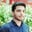Related Tags

pandas

# How to use the DataFrame.combine() method in pandasSalman Yousaf

### Overview

A Python library, pandas, is specially used for data manipulation and analysis. It provides multiple built-in methods for manipulating tables into DataFrames. In this shot, we are going to discuss the combine() method from the DataFrame module of this library.

Pandas logo

The DataFrame.combine() method is used to combine a DataFrame with the other DataFrame by using func to get element-wise combined columns. The dimensions of the resulting DataFrame will be the union of these DataFrames.

### Syntax

DataFrame.combine(other, func, fill_value=None, overwrite=True)

### Parameters

It takes the following argument values.

• other: Another DataFrame to combine element-wise.
• func: A function that takes two pandas series as inputs and returns a scaler series. It helps to combine two DataFrames.
• fill_value=None: The value to fill empty or NaNs before merging two columns. Its default value is None.
• overwrite=True: If overwrite is set to True, columns in self will be overwritten with NaNs in other. Its default value is True.

### Return value

The pandas DataFrame returns a combination of two self and other DataFrames.

### Explanation

In this code snippet, we are going to elaborate on how the combine() method can be used in different scenarios.

# load pandas module in program
import pandas as pd
# creating two DataFrames
df1 = pd.DataFrame({'A': [1,2,3], 'B': [0,-1,4]})
df2 = pd.DataFrame({'A': [0,2,1], 'B': [-3,4,9]})
smaller = lambda s1, s2: s1 if s1.sum() < s2.sum() else s2
df1.combine(df2, func=smaller)
print(df1)
# creating another two dataframes to check the functionality of fill_value
df3 = pd.DataFrame({'A': [0, 0], 'B': [None, 4]})
df4 = pd.DataFrame({'A': [1, 1], 'B': [3, 3]})
df1.combine(df4, smaller, fill_value=-5)
print(df3)

### Explanation

• Lines 1–5: We import the pandas library as pd in the program. In lines 4 and 5, we create two data frames df1 and df2.
• Line 6: We define a lambda function used to broadcast input values. It returns s1 if s1.sum() < s2.sum(). Otherwise, it returns s2.
• Line 7: We invoke pandas.combine() to merge two DataFrames, such as df1 & df2.
• Lines 10 and 11: We create new DataFrames df3 and df4.
• Line 12: We then invoke pandas.combine() to merge df3 and df4. It's taking df4, smaller, and fill_value=-5 as arguments. In case of missing values, it will be replaced with -5.

RELATED TAGS

pandas

CONTRIBUTORSalman Yousaf
RELATED COURSES

View all Courses

Keep Exploring

Learn in-demand tech skills in half the time